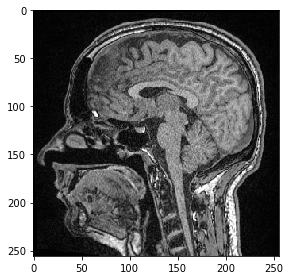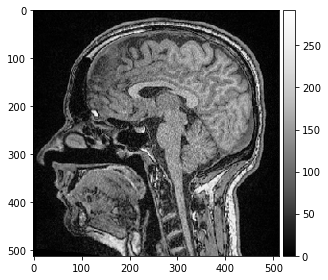# Affine transforms using cupy#

Here we apply an affine transform using cupy.

from skimage.io import imread, imshow

import cupy
from cupyx.scipy import ndimage as ndi

image = imread('../../data/Haase_MRT_tfl3d1.tif')

imshow(image)
image.shape

(192, 256, 256)Analogously to transforms in scipy also cupy used transform matrices to describe the transformation.

import numpy as np

# scaling by factor 1 / s
s = 0.5
matrix = np.asarray([
[s, 0, 0, 0],
[0, s, 0, 0],
[0, 0, s, 0],
[0, 0, 0, 1],
])


Before we can apply a cupy operation to an image, we need to send it to GPU memory. We receive a handle to an image on the GPU that cannot be shown using imshow.

cuda_image = cupy.asarray(image)
cuda_image.shape

(192, 256, 256)


Analogously to the affine transform in scipy, we need to create the output image with a defined shape, twice as large as the original image, before we can write into it.

output_shape = tuple((np.asarray(image.shape) / s).astype(int))
cuda_scaled = cupy.ndarray(output_shape)
print(cuda_scaled.shape)

(384, 512, 512)

r = ndi.affine_transform(cuda_image, cupy.asarray(matrix), output=cuda_scaled, output_shape=output_shape)


Before we can view the result image, we need to transfer it from GPU memory to CPU memory

result = cupy.asnumpy(cuda_scaled)
result.shape

(384, 512, 512)

imshow(result, cmap="Greys_r", vmin=0)

<matplotlib.image.AxesImage at 0x1d63076bdc0>